• 直接选择、二分法、冒泡、基数等排序方法都是稳定的排序方法。 5000个无序的元素，希望用最快的速度挑选出其中前50个最大的元素，最好选用堆排序。 栈和队列都只允许在端点处插入和删除元素。 深度...


直接选择、二分法、冒泡、基数等排序方法都是稳定的排序方法。

5000个无序的元素，希望用最快的速度挑选出其中前50个最大的元素，最好选用堆排序。

栈和队列都只允许在端点处插入和删除元素。

深度优先遍历是一个递归算法，在遍历的过程中，先访问的点被压入栈底。
答案A

不稳定：简单选择排序，希尔排序，快速排序，堆排序，冒泡 稳定：直接插入排序，归并排序，基数排序
转载于:https://www.cnblogs.com/pizishui/p/5347095.html
展开全文• 排序算法分为两种，稳定的和不稳定的，假设有下列一串数据：7,2,8,4,9,8。该数据有两个8，这里使用粗细来标记。对于稳定的排序，排序后的结果两个8不会互换位置，即2,4,7,8,8,9。 但是对于不稳定的排序，排序...
961全部内容链接
文章目录排序的基本概念插入排序直接插入排序折半插入排序希尔(Shell)排序
排序的基本概念
排序，就是重新排列列表中的元素，使表中的元素满足按关键字有序的过程。
排序算法分为两种，稳定的和不稳定的，假设有下列一串数据：7,2,8,4,9,8。该数据中有两个8，这里使用粗细来标记。对于稳定的排序，排序后的结果中两个8不会互换位置，即2,4,7,8,8,9。 但是对于不稳定的排序，排序之后，两个8的顺序就“有可能”发生变化，如2,4,7,8,8,9
根据数据是否全部存放于内存，还可以把排序分为两种：

内部排序：内部排序指的是要排序的数据比较小，可以直接全部存放于内存中
外部排序：相对于内部排序，要排序的数量比较大，比如几个G甚至几个T，无法直接存放于内存中，所以一次只能将一部分数据放入内存。这种排序称为外部排序。

插入排序
插入排序的基本思想：将待排序的序列分为两部分，前面是已经排好序的，后面是未排序的。每次从未排序的序列中选出一个，插入到已经排好序的序列。直到所有未排序序列为空。
直接插入排序
根据插入排序的思想，可以很容易的想到直接排序算法。

有序[ 0, …i ]
i + 1 (待排序元素)
i + 2，…，n (无序数列)

直接插入排序思想，第一部分为有序序列，第二部分是无序序列的第一个元素，第三部分是剩下的无序数列。算法（从小到大排列）如下：

从数组下标1开始进行循环，来选择待排序元素x
使x与其前面的一个元素进行对比，若比前面的元素小，则交换两个元素，否则说明x已经到了它想到的位置
重复2过程，直到x到了它应该到的地方
待1过程循环完毕，即排序完毕，该序列有序。

Java代码如下：
public static void insertionSort(Comparable[] array) {
if (array == null) return;

for (int i = 1; i < array.length; i++) {  // 从1位置开始，依次将后面的无序序列都插入到前面的有序序列

Comparable temp = array[i];
int j;
// 从最后一个开始比较，若已经比较到了0这个位置，或前面的元素比temp小，则说明此轮已经排序完成。
for (j = i; j > 0 && temp.compareTo(array[j-1]) < 0; j--) {
array[j] = array[j - 1];  // 这里之所以没有使用交换，是因为下一轮前面的元素又得把j-1给替换掉，所以统一在循环结束进行交换。
}
array[j] = temp; // 此时j就是要插入的位置，插入即可
}
}

复杂度分析

时间复杂度：平均时间复杂度和最坏的时间复杂度为O(n2)，这个应该没啥好说的，两层for循环。  最好的时间复杂度为O(n)，这个是因为当要排序的序列近乎有序时，每轮的比较都发现前一个元素已经比自己小了，这样就可以继续进行下一轮了。最终产生很少的元素交换。所以最好的时间复杂度为O(n)
空间复杂度：O(1)

稳定性：稳定的。每次比较时，只有前面的元素比后面的元素大时，才会发生交换，若相等则不会交换，所以是稳定的。 但是出题的时候可能会变成大于等于都会交换，此时就会是不稳定的。
适用性：此方式适用于顺序存储（数组）和链式存储。因为不存在随机访问。
折半插入排序
与直接插入排序差不多。直接插入排序是边比较边交换，而折半插入排序是：先对前面的有序数列进行折半查找，找出要插入的位置，然后再统一交换。其实效率差不多。但是折半插入不适用于链式存储。
代码如下：
TODO，非重点，后期补充
希尔(Shell)排序
希尔排序也是利用了直接插入的特性（近乎有序的序列时间复杂度O(n)）。基本思想为：

将序列分成若干组，然后对每组进行直接插入排序。
然后减小组的数量，相当于增大每组元素的个数，然后再次进行直接插入排序
重复2，当只剩一下一组时，即该组代表整个数组，进行最后一次排序，然后排序就完成了。

希尔排序难理解的地方就是这个分组，它并不是将相邻的元素分成一组。而是将相距固定距离的元素分为一组，如将下标 0,3,6,9…的元素分为一组，相应的1,4,7,11…分为一组。此时距离d=3。用专业的话就是：先将待排序的表分割成若干型如 L[i, i+d, i+2d, … , i+kd]的“特殊”子表，即把相隔某个“增量”的记录组成一个子表，对各个子表分别进行直接插入排序，当整个表中的元素已呈“基本有序”时，再对全体记录进行一次直接插入排序。
对于增量d，每轮都要进行递减。推荐的方式是，第一次取n/2，第二次取 n/2/2，每次除以2，直到为0。 但是出题时往往会给出不同的d的递减方式，来让你求每轮的排序结果。
举例：

9
6
7
4
1
6
2
9
3

第一轮
d=4
1
6
2
4
3
6
7
9
9

第一轮
d=4的组号
1 (1)
6 (2)
2 (3)
4 (4)
3 (1)
6 (2)
7 (3)
9 (4)
9 (1)

第二轮
d=2
1
4
2
6
3
6
7
9
9

第二轮
d=2的组号
1(1)
4(2)
2(1)
6(2)
3(1)
6(2)
7(1)
9(2)
9(1)

第三轮
d= 1
1
2
3
4
6
6
7
9
9

该例子中，经过了三轮将该序列排为有序序列。其中组号那一行的括号中相同的代表被分到了一组。考试中不需要单独画出分组情况。这里只是为了方便理解。
用java实现，代码如下：
public static void shellSort(Comparable[] array) {
if (array == null) return;

for (int d = array.length / 2; d > 0; d = d / 2) { // 第一轮 d=n/2,第二轮d=n/2/2，依次类推，直到d=0结束

for (int i = d; i < array.length; i++) {  // i取d，类似直接插入排序中的i=1
Comparable temp = array[i];  // 记录第一个位置的元素，用于该组交换过后的赋值
int j;
for (j = i; j >= d && temp.compareTo(array[j - d]) < 0; j = j - d) {  // 让当前元素与其 -kd 的元素对比，然后进行插入
array[j] = array[j - d];
}
array[j] = temp;
}
}
}

注意这里的第二个for循环。希尔排序虽然将其分成了若干组，但实际执行的时候，并不是将第一组排序完成之后才进行第二组排序。 而是先对第1组的第1个元素进行插入排序，然后对第2组的第1个元素进行排序，然后第3组的第1个，依次类推。然后是第1组的第二个元素…
复杂度分析:

时间复杂度：最好的时间复杂度为O(n)，平均复杂度为O(n1.3)，最坏的时间复杂度为O(n2)
空间复杂度：空间复杂度O(1)

稳定性：不稳定。若两个相同的数据被分为了不同的组，则有可能顺序会被调换
适用性：只适用于顺序存储。因为要用到随机访问的特性。


展开全文• 非常简单的排序算法，时间复杂度为O(n2)，是稳定的排序算法 9．在下列算法中，（　）算法可能出现下列情况：在最后一趟开始之前，所有的元素都不在其最终的位置上。 A）堆排序 B）冒泡排序 C）插入排序 D）快速...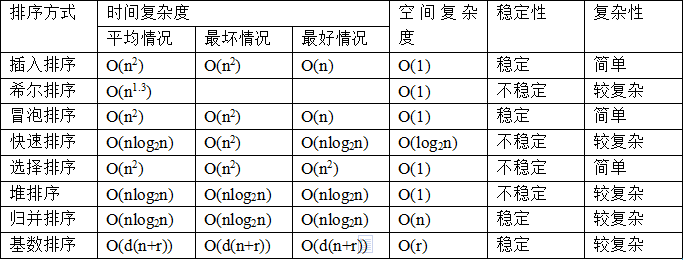插入排序
非常简单的排序算法，时间复杂度为O(n2)，是稳定的排序算法
9．在下列算法中，（　　）算法可能出现下列情况：在最后一趟开始之前，所有的元素都不在其最终的位置上。
A）堆排序    B）冒泡排序    C）插入排序    D）快速排序
【答案】C
【解析】在插入排序中，如果待排序列中的最后一个元素其关键字值为最小，则在最后一趟开始之前，前n-1个排好序的元素都不在其最终位置上，与排好序后的位置相差一个位置。因此，选C。

1 void insertsort(int a[], int n) {
2     for (int i = 2; i <= n; i++) {
3         if (a[i] < a[i - 1]){
4             a = a[i];
5             a[i] = a[i - 1];
6             int j;
7             for (j = i - 2; a[j] > a; j--) //比a大的都要往后移
8                 a[j + 1] = a[j];
9             a[j + 1] = a; //边界可以这么考虑，假设j=0了，也就是a最小，那么它应该放在a处，也就是a[j+1]
10         }
11     }
12 }

希尔排序
希尔排序是直接插入排序的一种改进算法，按照不同步长对元素进行插入排序，其时间复杂度与步长的选取有关，小于O(n2) ，如何选取步长增量序列是一个数学难题，当今还无人解决，是一种不稳定排序算法。
8．在文件“局部有序”或文件长度较小的情况下，最佳内部排序方法是（　　）
A）直接插入排序    B）冒泡排序    C）简单选择排序    D）归并排序
【答案】A
【解析】当待排序列基本有序时，对冒泡排序来说，若最大关键字位于序列首部，则每趟排序仅能使其“下沉”一个位置，要使其下沉到底部仍需n-1趟排序，也即时间复杂度仍为O(n2)。而对简单选择排序来说，其比较次数与待排序列的初始状态无关；归并排序要求待排序列已经部分有序，而部分有序的含义是待排序列由若干有序的子序列组成，即每个子序列必须有序，并且其时间复杂度为O(n log2n)；直接插入排序在待排序列基本有序时，每趟的比较次数大为降低，也即n-1趟比较的时间复杂度由O(n2)降至O(n)。
6．用希尔方法排序时，若关键字的初始排序杂乱无序，则排序效率就低（     ）
【答案】×
【解析】希尔排序又称“缩小增量排序”，即每趟只对相同增量距离的关键字进行比较，这与关键字序列初始有序或无序无关。

1 void shell(int a[], int n) {
2     int gap = n / 2;
3     while (gap >= 1) { // gap=1结束
4         for (int i = gap + 1; i <= n; i++) { // i=gap+1，从第一组的第二个元素开始往前插入排序
5             if (a[i] < a[i - gap]) {
6                 a = a[i];
7                 a[i] = a[i - gap];
8                 int j;
9                 for (j = i - gap ; j > 0 && a[j] > a; j = j - gap) //比a大的都要往后移动  j>0 这个条件很关键 否则容易越界，因为与直接插入排序不同
10                     a[j + gap] = a[j];
11                 a[j + gap] = a;
12             }
13         }
14         gap /= 2;
15     }
16 }

冒泡排序
冒泡排序比较简单粗暴，其时间复杂度为O(n2) ，是一种稳定的排序算法，两两元素互相比较并交换，每一趟选出最大的一个放在末尾

1 void bubble(int a[], int n)
2 {
3     for (int i = 1; i < n; i++) //n-1趟
4         for (int j = 1; j < n - i + 1; j++)
5             if (a[j] > a[j + 1]){
6                 int temp = a[j];
7                 a[j] = a[j + 1];
8                 a[j + 1] = temp;
9             }
10 }

快速排序
快速排序是目前被认为最好的一种排序方法，平均情况下快速排序的时间复杂度是Θ(nlogn)，最坏情况是Θ(n^2)。当划分产生的两个子问题分别包含 n-1 和 0 个元素时，最坏情况发生。划分操作的时间复杂度为Θ(n)，T(0)=Θ(1)，这时算法运行时间的递归式为 T(n)=T(n−1)+T(0)+Θ(n)=T(n−1)+Θ(n)，解为T(n)=Θ(n2)。当划分产生的两个子问题分别包含⌊n/2⌋和⌈n/2⌉−1个元素时，最好情况发生。算法运行时间递归式为 T(n)=2T(n/2)+Θ(n)，解为T(n)=Θ(nlgn)。事实上只要划分是常数比例的，算法的运行时间总是O(nlgn)。 假设按照 9:1 划分，每层代价最多为 cn，递归深度为 log10/9n=Θ(lgn)，故排序的总代价为 O(nlgn)。
快速排序方法在（　　）情况下最不利于发挥其长处。

Ａ）要排序的数据量太大

Ｂ）要排序的数据中含有多个相同值

Ｃ）要排序的数据已基本有序

Ｄ）要排序的数据个数为奇数

【答案】Ｃ （基本有序时就变成了冒泡排序了）
17．n个元素进行快速排序的过程中，第一次划分最多需要移动（　　）次元素(包括开始将基准元素移到临时变量的那一次)。
A）n／2    B）n-1    C）n    D）n+l
【答案】D
【解析】移动次数最多的情况是对n-1个元素比较时都需移动，加上开始将基准元素移到临时变量以及由临时变量移至正确位置的二次，即共需n+1次，故选D。
12．设表中元素的初态是按键值递增的，若分别用堆排序、快速排序、冒泡排序和归并排序方法对其仍按递增顺序进行排序，则_____________最省时间，_____________最费时间。
【答案】（1）冒泡排序　　（2）快速排序
【解析】若初始序列已经有序，则冒泡排序仅需一趟(比较n-1次)；而快速排序则需n-1趟，其时间复杂度升至O(n2)。因此填：冒泡排序，快速排序。
6．对一个具有7个记录的文件进行快速排序，请问：
（1）在最好情况下需进行多少次比较？并给出一个最好情况初始排列的实例。
（2）在最坏情况下需进行多少次比较？为什么？并给出此时的实例。
【答案】
（1）在最好情况下，由于快速排序是一个划分子区间的排序，每次划分最好能得到两个长度相等的子表，设表的长度为n=2k-1，显然有，第一遍划分得到两个长度均为　 n/2 　的子表。第二遍划分得到4个长度均为 n/4 　的子表，以此类推，总共进行k=log2(n+1)遍划分，各子表的长度均为1时，此时排序结束。
由于n=7,k=3，在最好情况下，第一遍经过6次，可找到一个其基准是正中间的元素，第二遍分别对两个子表（此时长度为3）进行排序，各需要2次，这样就可将整个数据序列排序完毕，从而知7个数据的最好情况下需进行10次比较。如：4，7，5，6，3，1，2。
（2）在最坏情况下，若每次划分时用的基准，它的关键字值是当前记录中最大（或最小值），那么每次划分只能得到左子表（或右子表），子表长度只比原表减少了一个。因此，若初始排列的记录是按关键字递增或递减的，而所得的结果须为递减或递增排列的，此时快速排序就退化为与冒泡排序相似，而且时间复杂度为O(n2)，此时反而不快了。对于n=7的数据序列，显然最坏情况下的比较次数为21。例如：7，6，5，4，3，2，1。

int Partition(int a[], int low, int high) { //这个函数是用来划分的
a = a[low]; // a是用来暂存 枢轴 的 所以数组从 1 开始到 10;
int pivotloc = a[low];
while (low < high) {   //长度为1就不需要划分了
while (low < high && a[high] >= pivotloc) --high;    //a[high]大于等于枢轴才能往前移动  漏了=号会造成死循环
a[low] = a[high];
while (low < high && a[low] <= pivotloc) ++low;      //a[low]小于等于枢轴才能往后移动  漏了=号会造成死循环
a[high] = a[low];
}
a[low] = a; //这句别漏了 枢轴要填回去
return low;
}
void Qsort(int a[], int low, int high) {
if (low < high) {  //这句别漏了  递归出口
int pivotloc;
pivotloc = Partition(a, low, high);  //比pivotloc小的放左边 大的放右边
Qsort(a, low, pivotloc - 1);         //递归排序左边  中间的pivotloc就不用排了 是枢轴
Qsort(a, pivotloc + 1, high);        //递归排序右边
}
}
void QuickSort(int a[], int n) {
Qsort(a, 1, n); //数组从1开始到n结束
}

堆排序

10．设有5000个无序的元素，希望用最快速度挑选出其中前10个最大的元素，在以下的排序方法中，采用（　　）方法最好

A）快速排序   B）堆排序   C）基数排序

【答案】B

【解析】用堆排序最好，因为堆排序不需要等整个排序结束就可挑出前10个最大元素，而快速排序和基数排序都需等待整个排序结束才能知道前10个最大元素。
12．以下序列不是堆的是（　　）
A）100,85,98,77,80,60,82,40,20,10,66
B）100,98,85,82,80,77,66,60,40,20,10
C）10,20,40,60,66,77,80,82,85,98,100
D）100,85,40,77,80,60,66,98,82,10,20
【答案】D
【解析】根据堆采用完全二叉树的顺序存储形式及堆的特点，因第一个结点即根结点关键字值最大，则应建立一个大根堆，但依据此数据序列建立起堆后关键字值为40的左右孩子结点分别为60、66，不符合大根堆特点。
堆不是二叉排序树，只要满足父节点比孩子节点大就行了（最大堆）
14．一组记录的关键字为{45，80，55，40，42，85}，则利用堆排序的方法建立的初始堆为（　　）
A）80,45,50,40,42,85
B）85,80,55,40,42, 45
C）85,80,55,45,42,40
D）85,55,80,42,45,40
【答案】B
7．对于关键字序列(12，13，11，18，60，15，7，20，25，100)，用筛选法建堆，必须从键值为_____________的关键字开始。
【答案】60
【解析】建堆必须从n／2结点开始，而10／2=5位置的结点值为60，故填60。
1．对一个堆，按二叉树层次进行遍历可以得到一个有序序列（     ）
【答案】×
【解析】堆的定义只规定了结点与其左、右孩子结点间的大小关系，而同一层上属不同父母的结点之间并无明确的大小关系，所以堆的层次遍历并不能得到一个有序序列。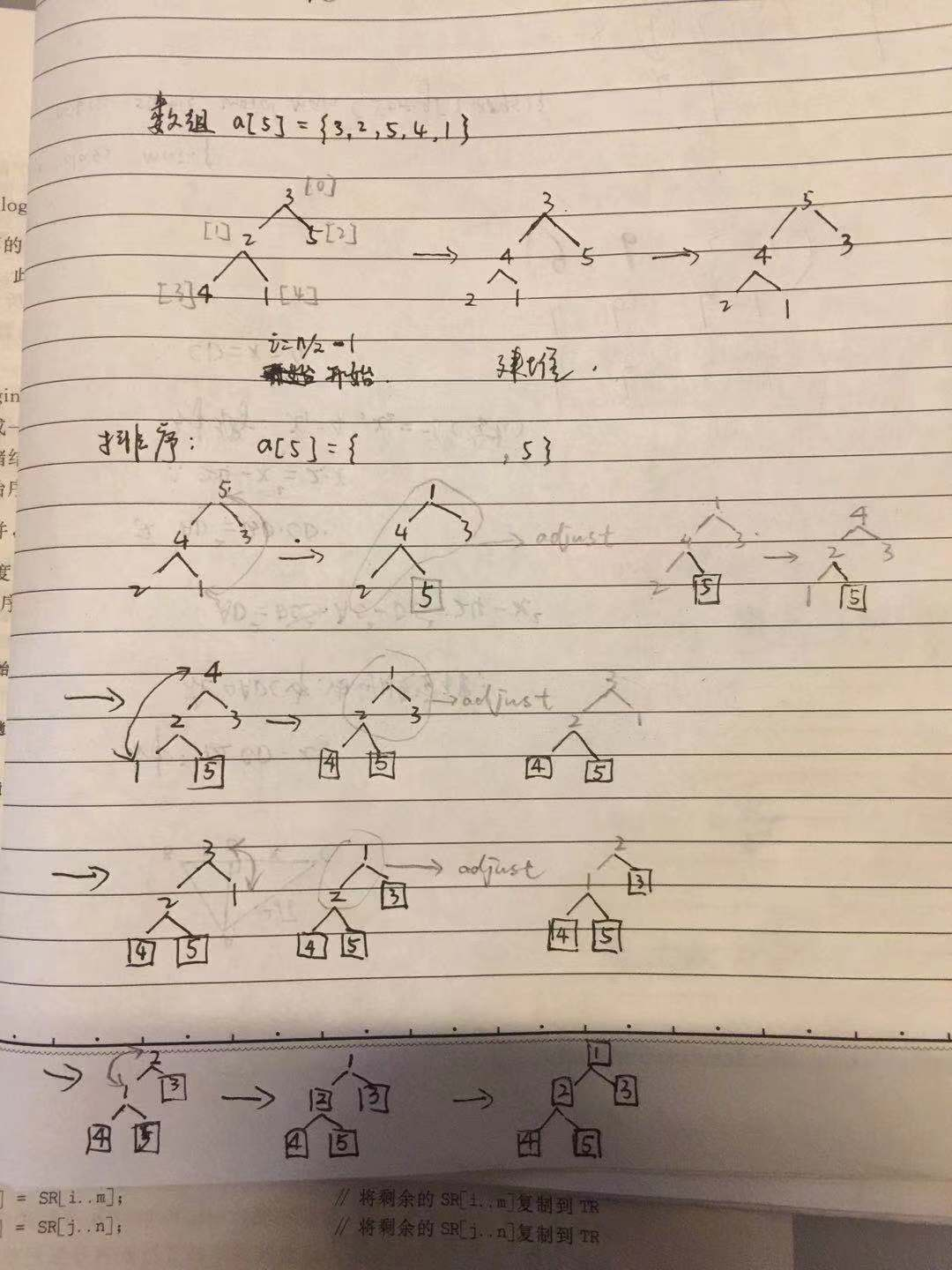void sift(int arr[],int low,int high)
{
int i=low,j=2*i+1;
int temp=arr[i];//用来暂时存储要修改的元素
while(j<=high)
{
if(j<high && arr[j]<arr[j+1])
++j;//在i所指节点有左右孩子的情况下找左右孩子中较大的
if(temp<arr[j]) //小的放到下面去
{
arr[i]=arr[j];
i=j;
j=2*i+1;
}
else
break;
}
arr[i]=temp;
}
void heatsort(int arr[],int n)
{
int i,temp;
//建立最大堆
for(i=n/2-1; i>=0; i--)
sift(arr,i,n-1);
for(i=n-1;i>=0;i--)
{
temp=arr;
arr=arr[i];
arr[i]=temp;
sift(arr,0,i-1);
}
}

2-路归并排序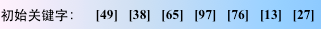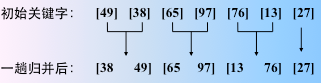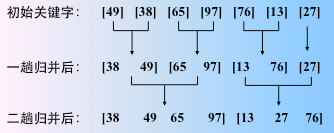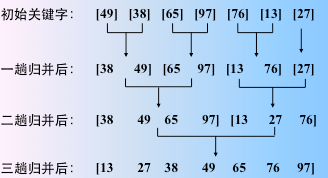对数组长度为n的序列进行归并排序，则大约要进行logn次归并，每一次合并都是线性时间O(n)。故粗略的计算出归并排序的时间复杂度是O(nlogn)(最好、最差都是这样)。空间复杂度是O(n)。归并排序是稳定的，并且是时间复杂度为o(nlogn)的几种排序(快速排序、堆排序)中唯一稳定的排序算法。

18．下述几种排序方法中，要求内存量最大的是（　　）
A）插入排序    B）选择排序    C）快速排序    D）归并排序
【答案】D
【解析】插入排序和选择排序需要的辅助空间为O(1)，快速排序需要的辅助空间为O(log2n )，归并排序需要的辅助空间为O(n)，因此选D。
14．在归并排序中，若待排序记录的个数为20，则共需要进行_____________趟归并，在第三趟归并中，是把长度为_____________的有序表归并为长度为_____________的有序表。
【答案】（1）5　（2）4　（3）8
【解析】第一次把长度为1的归并为长度的2的子表共10个，第二次把长度为2的归并成长度为4的子表共5个，第三次把长度为4的归并为长度为8的共3个，第四次长度为8归并为长度为16的，第5次归并成一个有序表。

//递归实现void merge(int a[], int b[], int first, int mid, int last)
{
int i, j, k;
i = first; j = mid + 1;
for (k = 0; i <= mid && j <= last; k++)
{
if (a[i] < a[j])  b[k] = a[i++];
else  b[k] = a[j++];
}
while (i <= mid) b[k++] = a[i++];
while (j <= last) b[k++] = a[j++];
for (i = 0; i < k; i++)
a[first + i] = b[i]; //不是让a从头开始赋值，是让a的某一个划分块被赋予b
}
void mergesort(int a[], int b[], int first, int last)
{
if (first < last)
{
int mid = (first + last) / 2;
mergesort(a, b, first, mid);
mergesort(a, b, mid + 1, last);
merge(a, b, first, mid, last);
}
}
int main()
{
int *a = new int{ 3,4,2,1,6 };
int *b = new int;
mergesort(a, b, 0, 4);
for (int i = 0; i < 5; i++)
cout << a[i] << " ";
cout << endl;
return 0;
}

//非递归
void Merge(string *a,  int first, int second, int len)
{
//合并[first...first+len-1][secont...second+len-1]
string *b = new string[n];
int i = first, j = second;
int k = 0;
for (; i <first+len && j <second+len && j<n; k++)
{
if (a[i] > a[j])
b[k] = a[i++];
else
b[k] = a[j++];
}
while (i < first + len)
b[k++] = a[i++];
while (j < second + len && j< n)  //注意这个区间的判断，因为并不是能保证第二个区间的长度也是len
//比如 [4,3,2,1]  第一个区间长度是4 但是第二个是1啊 不加这个会越界
b[k++] = a[j++];
for (i = 0; i < k; i++)
a[first + i] = b[i];
}
void MergeSort(string *a,int first, int last)
{
int len = 1;
int one_start, two_start;//前后两个区间的起始
while (len <= n) {         //len=n就只有一个区间了
one_start = first, two_start = one_start + len;
while (1) {
if (two_start>=n)  //当没有第二个区间的时候这一趟就结束了
break;
Merge(a, one_start, two_start, len); //归并one two两个区间，区间长度为len
one_start = two_start + len;
two_start = one_start + len;
}
print(a, n);
len = len * 2;
}
}

6．对n个数据进行简单选择排序，所需进行的关键字间的比较次数为_____________，时间复杂度为_____________。
【答案】（1）n(n-1)/2   （2）O(n2)
每一趟选出最大（小）的一个，第一趟比较除自身以外的n-1个元素，第二趟n-2，……，最后一趟 0，全部相加 等差数列求和Sn=(a1+an)*n/2

转载于:https://www.cnblogs.com/Liu269393/p/10212238.html
展开全文• 在计算器科学与数学中，一个排序算法（英语：Sorting algorithm）是一种能将一串数据依照特定排序方式进行排列一种算法。最常用到排序方式是数值顺序以及字典顺序。有效的排序算法在一些算法（例如搜索算法与...
文章目录排序算法稳定性常用排序算法比较算法实现插入排序冒泡排序选择排序希尔排序归并排序快速排序方法一方法二三路快速排序堆排序方法一方法二
排序算法

在计算器科学与数学中，一个排序算法（英语：Sorting algorithm）是一种能将一串数据依照特定排序方式进行排列的一种算法。最常用到的排序方式是数值顺序以及字典顺序。有效的排序算法在一些算法（例如搜索算法与合并算法）中是重要的，如此这些算法才能得到正确解答。排序算法也用在处理文字数据以及产生人类可读的输出结果。基本上，排序算法的输出必须遵守下列两个原则：

输出结果为递增序列（递增是针对所需的排序顺序而言）
输出结果是原输入的一种排列、或是重组

虽然排序算法是一个简单的问题，但是从计算器科学发展以来，在此问题上已经有大量的研究。举例而言，冒泡排序在1956年就已经被研究。虽然大部分人认为这是一个已经被解决的问题，有用的新算法仍在不断的被发明。（例子：图书馆排序在2004年被发表）     ------[维基百科]

排序算法一览

项目
内容

理论储备
• 计算复杂性理论 • 大O符号 • 全序关系 • 列表 • 稳定性 • 比较排序 • 自适应排序  • 排序网络 • 整数排序

交换排序
• 冒泡排序 • 鸡尾酒排序 • 奇偶排序 • 梳排序 • 侏儒排序 • 快速排序 • 臭皮匠排序 • Bogo排序

选择排序
• 选择排序 • 堆排序 • 平滑排序 • 笛卡尔树排序 • 锦标赛排序 • 圈排序

插入排序
• 插入排序 • 希尔排序 • 伸展排序 • 二叉查找树排序 • 图书馆排序 • 耐心排序

归并排序
• 归并排序 • 梯级归并排序 • 振荡归并排序 • 多相归并排序 • 列表排序

分布排序
• 美国旗帜排序 • 珠排序 • 桶排序 • 爆炸排序 • 计数排序 • 比较计数排序 • 鸽巢排序 • 相邻图排序 • 基数排序 • 闪电排序 • 插值排序

并发排序
• 双调排序器 • Batcher归并网络 • 两两排序网络

混合排序
• 块排序 • Tim排序 • 内省排序 • Spread排序 • J排序

其他
• 拓扑排序 • 煎饼排序 • 意粉排序

稳定性

当相等的元素是无法分辨的，比如像是整数，稳定性并不是一个问题。
然而，假设以下的数对将要以他们的第一个数字来排序。
(4,1)(3,1)(3,7)(5,6)
在这个状况下，有可能产生两种不同的结果，一个是让相等键值的纪录维持相对的次序，而另外一个则没有：
(3,1)(3,7)(4,1)(5,6)  ------ 维持次序
(3,7)(3,1)(4,1)(5,6)  ------ 次序改变
不稳定排序算法可能会在相等的键值中改变纪录的相对次序，但是稳定排序算法从来不会如此。
稳定的排序

冒泡排序（bubble sort）— O(n2)
插入排序（insertion sort）— O(n2)
鸡尾酒排序（cocktail sort）— O(n2)
桶排序（bucket sort）— O(n)；需要 O(k) 额外空间
计数排序（counting sort）— O(n+k)；需要 O(n+k)额外空间
归并排序（merge sort）— O(n log n)；需要 O(n)额外空间
原地归并排序   — O(nlog2n)
二叉排序树排序（binary tree sort）— O(n log n)期望时间；O(n2)最坏时间；需要 O(n)额外空间
鸽巢排序（pigeonhole sort）— O(n+k)；需要 O(k) 额外空间
侏儒排序（gnome sort）— O(n2)
图书馆排序（library sort）— O(n log n)期望时间；O(n2)最坏时间；需要 (1+ε)n额外空间
块排序（block sort）— O(n log n)

不稳定的排序

选择排序（selection sort）— O(n2)
希尔排序（shell sort）— O(n log2n)
C - lover排序算法（Clover sort）— O(n)期望时间， O(n2)最坏情况
梳排序 — O(n log n)
堆排序（heap sort）— O(n log n)
平滑排序（smooth sort）— O(n log n)
快速排序（quick sort）— O(n log n)期望时间， O(n2)最坏情况；对于大的、随机数列表一般相信是最快的已知排序
内省排序（introsort）— O(n log n)
耐心排序（patience sort）— O(n log n+k) 最坏情况时间， O(n+k)空间，也需要找到最长的递增子序列（longest increasing subsequence）

不实用的排序

Bogo排序 — O(n * n!)，最坏的情况下期望时间为无穷。
Stupid排序 — O(n3);递归版本需要O(n2)额外存储器
煎饼排序 —  O(n),但需要特别的硬件
臭皮匠排序（stooge sort）算法简单，但需要约 n2.7 的时间

常用排序算法比较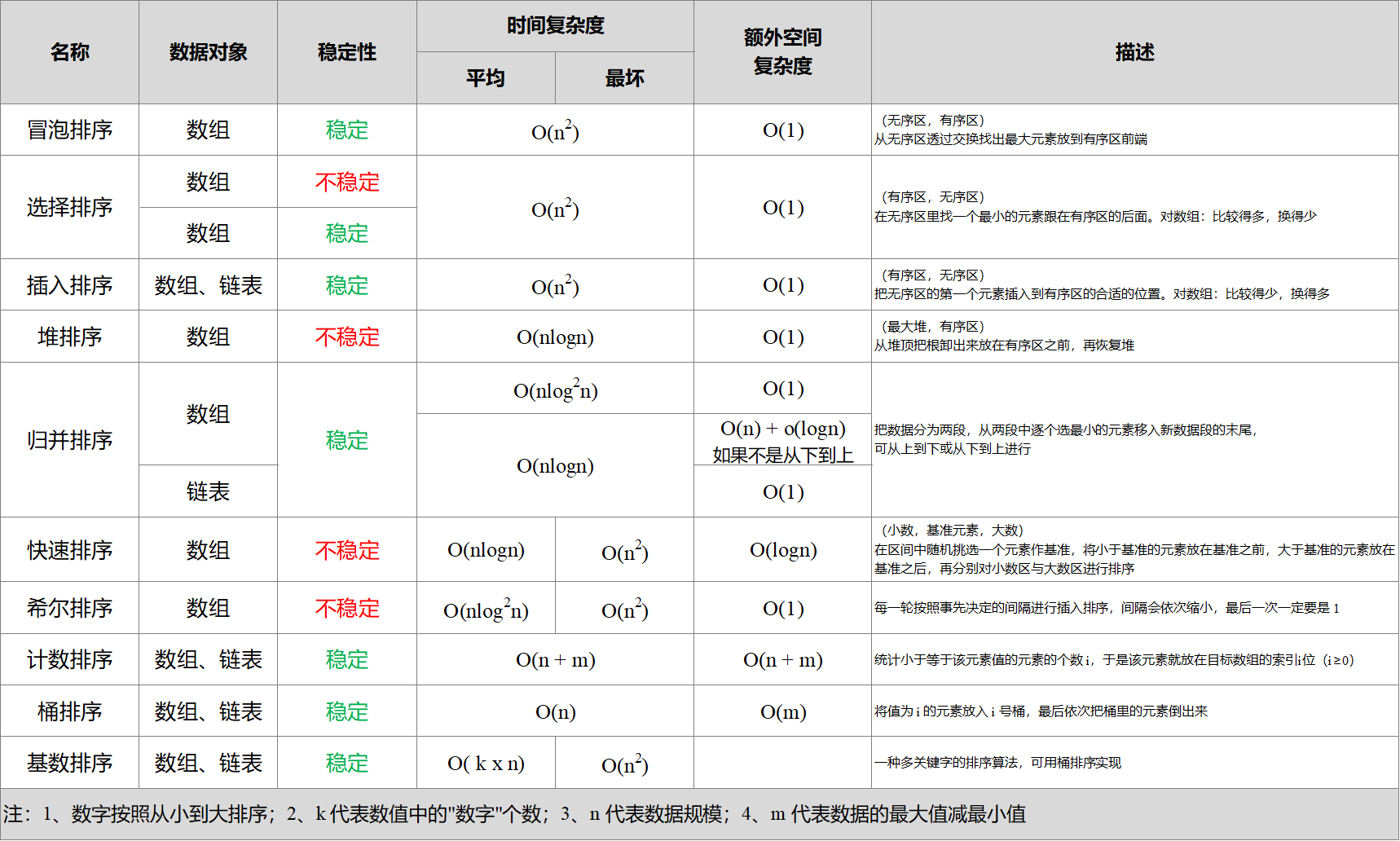算法实现

插入排序
// 一般方法
void insertSort(int array[], int arraySize) {
int i, j;
int tmp;

for(i = 1; i < arraySize; i++) {
tmp = array[i];
j = i - 1;
while(j >= 0 && tmp > array[j]) {           /*从大到小排序，因此改变判断条件*/
array[j + 1] = array[j--];
}
array[j + 1] = tmp;                     /*将元素tmp插入指定位置*/
}
}

// 模板方法
template<typename T>
void insertionSort(T arr[], int n){

for( int i = 1 ; i < n ; i ++ ) {

// 寻找元素arr[i]合适的插入位置
// 写法1
//        for( int j = i ; j > 0 ; j-- )
//            if( arr[j] < arr[j-1] )
//                swap( arr[j] , arr[j-1] );
//            else
//                break;

// 写法2
//        for( int j = i ; j > 0 && arr[j] < arr[j-1] ; j -- )
//            swap( arr[j] , arr[j-1] );

// 写法3
T e = arr[i];
int j; // j保存元素e应该插入的位置
for (j = i; j > 0 && arr[j-1] > e; j--)
arr[j] = arr[j-1];
arr[j] = e;
}
}

// 进阶
for (auto i = v.begin(); i != v.end(); ++i) {
std::rotate(std::upper_bound(v.begin(), i, *i), i, i + 1);
}

冒泡排序
// 一般方法
void bubbleSort(int array[],int arraySize)
{
int i,j,tmp ,flag = 1;
for(i=0;i<arraySize-1 && flag == 1;i++){       /*arraySize个元素的序列执行arraySize-1趟冒泡排序*/
flag = 0;								   /*flag初始化为0*/
for(j=0;j<arraySize-i;j++) {
if(array[j]<array[j+1]) {              /*数据交换,将较小的数据往后交换，实现从大到小排序*/
tmp = array[j+1];
array[j+1] = array[j];
array[j] = tmp;
flag = 1;						  /*发生数据交换，标志flag置为1*/
}
}
}
}

// 模板方法
template<typename T>
void bubbleSort( T arr[] , int n){

bool swapped;
//int newn; // 理论上,可以使用newn进行优化,但实际优化效果较差

do{
swapped = false;
//newn = 0;
for( int i = 1 ; i < n ; i ++ )
if( arr[i-1] > arr[i] ){
swap( arr[i-1] , arr[i] );
swapped = true;

// 可以记录最后一次的交换位置,在此之后的元素在下一轮扫描中均不考虑
// 实际优化效果较差,因为引入了newn这个新的变量
//newn = n;
}

//n = newn;

// 优化,每一趟Bubble Sort都将最大的元素放在了最后的位置
// 所以下一次排序,最后的元素可以不再考虑
// 理论上,newn的优化是这个优化的复杂版本,应该更有效
// 实测,使用这种简单优化,时间性能更好
n --;

}while(swapped);
}

选择排序
// 一般方法
void selectSort(int array[], int arraySize) {
int i, j, min, tmp;
for(i = 0; i < arraySize - 1; i++) {
min = i;
for(j = i + 1; j < arraySize; j++) { /*在未排序的子序列中找到最小的元素位置*/
if(array[j] < array[min]) {
min = j;          /*用min记录下最小元素的位置*/
}
}
if(min != i) {                    /*最小的元素不位于子序列的第1个位置*/
tmp = array[min] ;
array[min] = array[i];       /*元素的交换*/
array[i] = tmp;
}
}
}

// 模板方法
template<typename T>
void selectionSort(T arr[], int n){

for(int i = 0 ; i < n ; i ++){

int minIndex = i;
for( int j = i + 1 ; j < n ; j ++ )
if( arr[j] < arr[minIndex] )
minIndex = j;

swap( arr[i] , arr[minIndex] );
}
}

// 进阶
#include <algorithm>
#include <iterator>
template<class ForwardIt>
void selection_sort(ForwardIt begin, ForwardIt end)
{
for (ForwardIt i = begin; i != end; ++i)
std::iter_swap(i, std::min_element(i, end));
}

希尔排序
// 一般方法
void shellSort(int array[],int arraySize)
{
int i, j, flag ,gap = arraySize;
int tmp;
while(gap > 1){
gap = gap/2;                    /*按照经验值，每次缩小增量一半*/
do{                             /*子序列可以使用冒泡排序*/
flag = 0;
for(i=0;i<arraySize-gap;i++){
j = i + gap;
if(array[i]>array[j]) {        /*子序列按照冒泡排序方法处理*/
tmp = array[i];            /*交换元素位置*/
array[i] = array[j];
array[j] = tmp;
flag = 1;             /*设置标志flag*/
}
}
}while(flag !=0);                 /*改进了的冒泡排序法*/
}
}

// 模板方法
template<typename T>
void shellSort(T arr[], int n){

// 计算 increment sequence: 1, 4, 13, 40, 121, 364, 1093...
int h = 1;
while( h < n/3 )
h = 3 * h + 1;

while( h >= 1 ){

// h-sort the array
for( int i = h ; i < n ; i ++ ){

// 对 arr[i], arr[i-h], arr[i-2*h], arr[i-3*h]... 使用插入排序
T e = arr[i];
int j;
for( j = i ; j >= h && e < arr[j-h] ; j -= h )
arr[j] = arr[j-h];
arr[j] = e;
}

h /= 3;
}
}

归并排序
// 使用优化的归并排序算法, 对arr[l...r]的范围进行排序
template<typename T>
void __mergeSort2(T arr[], int l, int r){

// 优化2: 对于小规模数组, 使用插入排序
if( r - l <= 15 ){
insertionSort(arr, l, r);
return;
}

int mid = (l+r)/2;
__mergeSort2(arr, l, mid);
__mergeSort2(arr, mid+1, r);

// 优化1: 对于arr[mid] <= arr[mid+1]的情况,不进行merge
// 对于近乎有序的数组非常有效,但是对于一般情况,有一定的性能损失
if( arr[mid] > arr[mid+1] )
__merge(arr, l, mid, r);
}

template<typename T>
void mergeSort2(T arr[], int n){

__mergeSort2( arr , 0 , n-1 );
}

// 进阶

#include <vector>
#include <iostream>
#include <algorithm>

template<class Iter>
void merge_sort(Iter first, Iter last)
{
if (last - first > 1) {
Iter middle = first + (last - first) / 2;
merge_sort(first, middle);
merge_sort(middle, last);
std::inplace_merge(first, middle, last);
}
}

int main()
{
std::vector<int> v{8, 2, -2, 0, 11, 11, 1, 7, 3};
merge_sort(v.begin(), v.end());
for(auto n : v) {
std::cout << n << ' ';
}
std::cout << '\n';
}

快速排序
方法一
void swap(int *a, int *b) {
/*交换序列中元素的位置*/
int tmp;
tmp = *a;
*a = *b;
*b = tmp;
}

void quickSortArray(int array[], int s, int t) {
int low, high;
if(s < t) {
low = s;
high = t + 1;
while(1) {
do low++;
while(array[low] >= array[s] && low != t);    /*array[s]为基准元素，重复执行low++操作*/
do high--;
while(array[high] <= array[s] && high != s);  /*array[s]为基准元素，重复执行high--操作*/
if(low < high)
swap(&array[low], &array[high]);          /*交换array[low]和array[high]的位置*/
else
break;
}
swap(&array[s], &array[high]);                  /*将基准元素与array[high]进行交换*/
quickSortArray (array, s, high - 1);            /*将基准元素前面的子序列快速排序*/
quickSortArray (array, high + 1, t);            /*将基准元素后面的子序列快速排序*/
}
}

void quickSort(int array[], int arraySize) {
quickSortArray(array, 0, arraySize - 1);
}

方法二
// 对arr[l...r]部分进行partition操作
// 返回p, 使得arr[l...p-1] < arr[p] ; arr[p+1...r] > arr[p]
template <typename T>
int _partition(T arr[], int l, int r){

// 随机在arr[l...r]的范围中, 选择一个数值作为标定点pivot
swap( arr[l] , arr[rand()%(r-l+1)+l] );

T v = arr[l];
int j = l;
for( int i = l + 1 ; i <= r ; i ++ )
if( arr[i] < v ){
j ++;
swap( arr[j] , arr[i] );
}

swap( arr[l] , arr[j]);

return j;
}

// 双路快速排序的partition
// 返回p, 使得arr[l...p-1] < arr[p] ; arr[p+1...r] > arr[p]
template <typename T>
int _partition2(T arr[], int l, int r){

// 随机在arr[l...r]的范围中, 选择一个数值作为标定点pivot
swap( arr[l] , arr[rand()%(r-l+1)+l] );
T v = arr[l];

// arr[l+1...i) <= v; arr(j...r] >= v
int i = l+1, j = r;
while( true ){
// 注意这里的边界, arr[i] < v, 不能是arr[i] <= v
// 思考一下为什么?
while( i <= r && arr[i] < v )
i ++;

// 注意这里的边界, arr[j] > v, 不能是arr[j] >= v
// 思考一下为什么?
while( j >= l+1 && arr[j] > v )
j --;

// 对于上面的两个边界的设定, 有的同学在课程的问答区有很好的回答:)
// 大家可以参考: http://coding.imooc.com/learn/questiondetail/4920.html

if( i > j )
break;

swap( arr[i] , arr[j] );
i ++;
j --;
}

swap( arr[l] , arr[j]);

return j;
}

// 对arr[l...r]部分进行快速排序
template <typename T>
void _quickSort(T arr[], int l, int r){

// 对于小规模数组, 使用插入排序进行优化
if( r - l <= 15 ){
insertionSort(arr,l,r);
return;
}

// 调用双路快速排序的partition
int p = _partition2(arr, l, r);
_quickSort(arr, l, p-1 );
_quickSort(arr, p+1, r);
}

template <typename T>
void quickSort(T arr[], int n){

srand(time(NULL));
_quickSort(arr, 0, n-1);
}

三路快速排序
// 递归的三路快速排序算法
template <typename T>
void __quickSort3Ways(T arr[], int l, int r){

// 对于小规模数组, 使用插入排序进行优化
if( r - l <= 15 ){
insertionSort(arr,l,r);
return;
}

// 随机在arr[l...r]的范围中, 选择一个数值作为标定点pivot
swap( arr[l], arr[rand()%(r-l+1)+l ] );

T v = arr[l];

int lt = l;     // arr[l+1...lt] < v
int gt = r + 1; // arr[gt...r] > v
int i = l+1;    // arr[lt+1...i) == v
while( i < gt ){
if( arr[i] < v ){
swap( arr[i], arr[lt+1]);
i ++;
lt ++;
}
else if( arr[i] > v ){
swap( arr[i], arr[gt-1]);
gt --;
}
else{ // arr[i] == v
i ++;
}
}

swap( arr[l] , arr[lt] );

__quickSort3Ways(arr, l, lt-1);
__quickSort3Ways(arr, gt, r);
}

template <typename T>
void quickSort3Ways(T arr[], int n){

srand(time(NULL));
__quickSort3Ways( arr, 0, n-1);
}

堆排序
方法一
#include "stdio.h"

{
int j;
int tmp;
tmp = k[i-1];
j = 2 * i ;
while(j<=n)
{
if(j<n && k[j-1]>k[j])
{
j++;				/* j为i的左右孩子中较小孩子的序号*/
}
if(tmp<=k[j-1])
{
break;				/*tmp为最小的元素，则不需要元素的交换*/
}
k[j/2-1] = k[j-1];				/*交换元素位置*/
j = 2 * j ;
}
k[j/2-1] = tmp;
}

void heapSort(int k[],int n)
{
int i,j;
int tmp;
for(i=n/2;i>=1;i--)
{
}

for(i=n-1;i>=0;i--)
{
tmp = k[i];			/*调整序列元素*/
k[i] = k;
k = tmp;
}
}

main()
{
int i,k= {5,2,12,6,9,0,3,6,15,20};
printf("The orginal data array is\n") ;
for(i=0;i<10;i++)                        /*显示原序列之中的元素*/
printf("%d ",k[i]);
heapSort(k,10);                         	/*快速排序*/
printf("\nThe result of heap sorting for the array is\n");
for(i=0;i<10;i++)                       	/*显示排序后的结果*/
printf("%d ",k[i]);
getchar();
getchar();
}

方法二
template<typename Item>
class MaxHeap{

private:
Item *data;
int count;
int capacity;

void shiftUp(int k){
while( k > 1 && data[k/2] < data[k] ){
swap( data[k/2], data[k] );
k /= 2;
}
}

void shiftDown(int k){
while( 2*k <= count ){
int j = 2*k;
if( j+1 <= count && data[j+1] > data[j] ) j ++;
if( data[k] >= data[j] ) break;
swap( data[k] , data[j] );
k = j;
}
}

public:

// 构造函数, 构造一个空堆, 可容纳capacity个元素
MaxHeap(int capacity){
data = new Item[capacity+1];
count = 0;
this->capacity = capacity;
}

// 构造函数, 通过一个给定数组创建一个最大堆
// 该构造堆的过程, 时间复杂度为O(n)
MaxHeap(Item arr[], int n){
data = new Item[n+1];
capacity = n;

for( int i = 0 ; i < n ; i ++ )
data[i+1] = arr[i];
count = n;

for( int i = count/2 ; i >= 1 ; i -- )
shiftDown(i);
}

~MaxHeap(){
delete[] data;
}

// 返回堆中的元素个数
int size(){
return count;
}

// 返回一个布尔值, 表示堆中是否为空
bool isEmpty(){
return count == 0;
}

// 像最大堆中插入一个新的元素 item
void insert(Item item){
assert( count + 1 <= capacity );
data[count+1] = item;
shiftUp(count+1);
count ++;
}

// 从最大堆中取出堆顶元素, 即堆中所存储的最大数据
Item extractMax(){
assert( count > 0 );
Item ret = data;
swap( data , data[count] );
count --;
shiftDown(1);
return ret;
}

// 获取最大堆中的堆顶元素
Item getMax(){
assert( count > 0 );
return data;
}
};

// heapSort1, 将所有的元素依次添加到堆中, 在将所有元素从堆中依次取出来, 即完成了排序
// 无论是创建堆的过程, 还是从堆中依次取出元素的过程, 时间复杂度均为O(nlogn)
// 整个堆排序的整体时间复杂度为O(nlogn)
template<typename T>
void heapSort1(T arr[], int n){

MaxHeap<T> maxheap = MaxHeap<T>(n);
for( int i = 0 ; i < n ; i ++ )
maxheap.insert(arr[i]);

for( int i = n-1 ; i >= 0 ; i-- )
arr[i] = maxheap.extractMax();

}

// heapSort2, 借助我们的heapify过程创建堆
// 此时, 创建堆的过程时间复杂度为O(n), 将所有元素依次从堆中取出来, 实践复杂度为O(nlogn)
// 堆排序的总体时间复杂度依然是O(nlogn), 但是比上述heapSort1性能更优, 因为创建堆的性能更优
template<typename T>
void heapSort2(T arr[], int n){

MaxHeap<T> maxheap = MaxHeap<T>(arr,n);
for( int i = n-1 ; i >= 0 ; i-- )
arr[i] = maxheap.extractMax();

}



展开全文• 一、排序介绍排序算法有很多，比较基础下列10种：冒泡排序选择排序插入排序希尔排序归并排序快速排序堆排序计数排序桶排序基数排序排序算法还可以根据其稳定性，划分为稳定排序和不稳定排序。即如果值相同元素...
• 这个时候就要用到一种新的排序方法——插入排序法,插入排序的基本操作就是将一个数据插入到已经排好序有序数据中，从而得到一个新、个数加一有序数据，算法适用于少量数据的排序，时间复杂度为O(n^2)。...
• 2. 在下列排序方法中，稳定的方法有（） A. 归并排序与基数排序 B. 插进排序与希尔排序 C. 堆排序与快进排序 D. 选择排序与冒泡排序 答：C 3. 一个递归算法包括（） A. 递归部分 B. 终止条件和递归部分 C. 迭代...
• 1、在下列四种排序算法，只有（ ）是一种不稳定排序 A、冒泡排序 B、选择排序 C、插入排序 D、归并排序 2、一个数组，含有大量重复元素，使用（ ）进行排序是一种合理抉择 A、快速排序 B、双路快速排序 C、三路...
• 1、快速排序：最好是...5、下列排序算法中，某一趟排序结束后未必能选出一个元素放在棋最终位置上的是（） 正确答案: C 堆排序 冒泡排序 直接插入排序（不行） 快速排序 6、外部排序常用的算法是？ 正确答案: A 归并
• 下列排序方法中，稳定的排序算法是______。 A）冒泡排序 B）归并排序 C）快速排序 D）直接插入排序 \4. 快速排序在最坏情况下的时间复杂度为_____。 A）O( 1 ) B）O( n ) C）O( n log2n ) D） O( n2 ) 8.下列排序...
• 第7章 排序 一、选择题 1．某内排序方法的稳定性是指( D )。 A．该排序算法不允许有相同的关键字记录B．...C．...2．下面给出的四种排序法中( D )排序法是不...3．下列排序算法中，其中（ D ）是稳定的。 A. 堆排...
• u导读：　C．平均时间为0（n log n）的排序方法 D．以上都不对 2．下面给出的四种排序法中( )排序法是不稳定性排序法。【北京航空航天大学1999 一、10 ...下列排序算法中，其中（ ）是稳定的。 【福州大学1998 一、3integer list pascal
• 10．下列排序算法中，稳定的排序算法是（ ）。 A．堆排序 B．直接插入排序  C．快速排序 D．希尔排序 11．堆排序的时间复杂度是（ ）。 A．O(n*n) B．O(n*log n)  C．O(n) D．O(log n) 填空题（每空4分，共4...
• 概念： 堆： 堆（英语：heap)是计算机科学...堆排序是利用堆这种数据结构而设计一种排序算法，堆排序是一种选择排序，它最坏，最好，平均时间复杂度均为O(nlogn)，它也是不稳定排序。首先简单了解下堆结构。 满足
• 1、选择题(1)直接插入排序算法的时间复杂度为（ ）A．O（N） B.O（1） C.O(N2) D.O(LOGN)(2)下列排序方法中，从平均时间而言最佳的是（ ）A．快速 B.希尔 C.基数 D.归并(3)下列是稳定的排序方法的（ ）A．快速 B....
• ## 算法导论测试

千次阅读 2020-03-25 12:09:16
下列四种排序方法，在排序中关键字比较次数同记录初始排列无关的是( )。 A、直接插入 B、堆排序 C、快速排序 D、归并排序 正确答案： D 3 稳定的排序方法是（ ） A、直接插入排序和快速排序 B、折半插入排序和冒泡...算法 数据结构 快速排序 大数据
• (排序预习题) 下列排序算法中，（）是稳定的。 ① 插入排序 ② 快速排序 ③ 堆排序 ④ 归并排序 2021-05-04 22:58:30 A、 ①和② B、 ②和③ C、 ③和④ D、 ①和④ 回答正确正确答案:D 解析: ①和④ 附...
• 第８章 排序 本章中主要介绍下列内容：　1.插入排序　2.交换排序　3.选择排序　4....如果排序依据的是主关键字，排序的结果将是唯一的， 排序算法稳定性：如果在待排序的记录序列有多个数据结构 exchange 存储 null
• 下列排序算法中平均时间复杂度是 O(nlogn)且稳定的是？ （ ） A. 冒泡排序 B. 插入排序 C. 归并排序 D. 堆排序 E. 快速排序 2.经典的 OSI 模型定义了一个七层网络协议，下列协议中属于网络层的是?（） A. IP B. TCP ...
• 下列排序算法中其中 是稳定的 A.堆排序冒泡排序 B.快速排序堆排序 C.直接选择排序归并排序 D.归并排序冒泡排序 2.若需在Onlog2n的时间内完成对数组的排序且要求排序是稳定的贝U可选 择的排序方法是 A.快速排序 B.堆...
• 说明: 在 SQL 语句中, 应避免使用繁琐 NLS_SORT 进程。正常情况下, WHERE 子句中进行比较是二进制, 但语言比较则需要 NLSSORT 函数。可以使用 NLS_COMP 指定必须根据NLS_SORT 会话参数进行语言比较。 值范围:...
• 下列排序算法中稳定且时间复杂度为O(n2)的是（冒泡排序） 2. 在分块查找中，若索引表各块内均用顺序查找，则有324个元素线性表若分成9块，其平均查找长度为（23.5） 324个元素，分成9块，每块36个元素。...
• 5．（4分）给出拓扑排序算法描述（不用写C/C++算法） 四、算法设计（用类-C/类-C++描述）（16分） 1．(8分)完成一个二叉树左右子树交换递归算法。 2．(8分)设在一个带头结点双向链表中，所有结点数据元素按值...
• 下一节排序中，有序含义也是如此。 对于长度为n有序线性表，利用二分法查找元素X过程如下： 步骤1：将X与线性表中间项比较； 步骤2：如果X值与中间项值相等，则查找成功，结束查找； 步骤3：如果X小于...
• [注]：编写程序可选用任一种高语言，...4、 哈希方法基本思想5、 给出一不稳定排序方法名称与实例二、 构造结果：[24分](1) 确定x: x+1语句在下面程序段中的频率，要求写出分析过程。for i: 1ton doforj: 1toI d...
• 10、在内部排序中，排序时不稳定的是： （10） 。 （10） A. 插入排序 B. 冒泡排序 C. 快速排序 D. 归并排序 11、在含有n个结点的树中，边的数目只能是 （11） 条。 （11） A. n B. n*(n-1) C. n-1...
• 15. 下列数据中，（C ）是非线性数据结构。【北京理工大学 2001 六、1（2分）】 A．栈 B. 队列 C. 完全二叉树 D. 堆 16．连续存储设计时，存储单元地址（A ）。【中山大学 1999 一、1（1分）】 A．一定连续 B．...
• 12.在最好和最坏情况下的时间复杂度均为O(nlogn)且稳定的排序方法是( ) A.快速排序 O(n*n) B.堆排序 C.归并排序 D.基数排序 13.不可能生成右图所示二叉排序树的关键字序列是( ) A.4 5 3 1 2 B.4 2 5 3 1 C.4 5 2...数据结构题